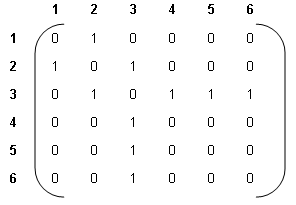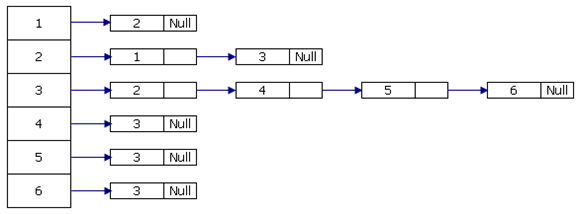## Show the linked representation of the graph, Physics

Assignment Help:

Q. Show the linked representation of the graph.Ans: The linked representation of given graph is:#### Determine the bending moment, Determine the Shear Force in kN rounded to 1 ...

Determine the Shear Force in kN rounded to 1 DP and Bending Moment in kN.m rounded to 1 DP at point C in the beam when the UDL on the beam is P52 kN/m (as for the previous question

#### Explain centers of the central band and the first-order band, A physics stu...

A physics student performs a double-slit diffraction experiment on an optical bench, as shown in the figure below. The light from a helium-neon laser has a wavelength of 632.8

#### Which series describes electron transition for hydrogen atom, For the hydro...

For the hydrogen atom, which series describes electron transitions to the N=1 orbit, the lowest energy electron orbit? Is it the: a)  Lyman series b)  Balmer series

#### Evaluate the natural frequency of the body, The differential equation of ...

The differential equation of motion of freely oscillating body is given by 2 d 2 x/dt 2 + 8π 2 x = 0. Evaluate the natural frequency of the body?

#### Characterictic graph of diode, for silicon and germinium

for silicon and germinium

#### Optical fibre, What are the types of fibre optics?

What are the types of fibre optics?

#### Potential difference, The voltage across the terminals of a cell when it is...

The voltage across the terminals of a cell when it is supplying current to external resistance is known as potential difference or final voltage. Potential energy dissimilarity is

#### Heat equation + matlab, I need to solve and write a MATLAB code the heat eq...

I need to solve and write a MATLAB code the heat equation by two methods ( Crank-Nicolson method and asymptotic method )

#### Explain the electromagnetic induction, Explain the Electromagnetic Inductio...

Explain the Electromagnetic Induction The force exerted on an electric charge moving in a magnetic field leads to the idea of magnets being able to produce electricity as well